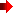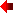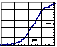# Enzyme Kinetics

Michaelis-Menton (MM) KineticsExtended MM equation for interacting sites
 [E] + [S]
 k1k2
 [ES]
 k3[E] + [P]
Assumptions and givens:
• d[ES]/dt = 0 -- steady-state approximation
• [P] = 0 -- at time zero
• V = d[P]/dt = k3 [ES]
• [Etotal] = [E] + [ES]
• Vmax = k3 [Etotal]
• Km = (k2+ k3)/k1= [S]1/2
where V = (1/2) Vmax
V / Vmax = [S] / ([S] + Km ) -- MM equation
 [E] + n [S]
 k1k2
 [ESn]
 k3[E] + n[P]
Assumptions and givens:
• d[ESn]/dt = 0 -- steady-state approximation
• [P] = 0 -- at time zero
• V = d[P]/dt = n k3 [ESn]
• [Etotal] = [E] + [ESn]
• Vmax = n k3 [Etotal]
• Km = (k2+ k3)/k1= ([S]1/2)n
where V = (1/2) Vmax
V / Vmax = [S]n / ([S]n + Km )
where n = Hill coefficient

Graphical Methods
Rectangular hyperbolic plot
(n = Hill coefficient)
Double-reciprocal plot
"Ya" = V / Vmax = [S]n / ([S]n + Km )
where n = 1 for a MM enzyme
Vmax / V = 1 + Km / [S]n
where n = 1 for a MM enzyme
Hill plotScatchard plot
V/( Vmax - V ) = "Ya"/"Yd" = [S]n / Km
where n = 1 for a MM enzyme and
"Yd" = (Vmax - V ) / Vmax = Km / ( [S]n + Km )
(V / Vmax) / [S]n = ( 1 / Km ) * (1 - V / Vmax)
where n = 1 for a MM enzyme
Direct linear plot (n = 1)
Vmax = V + ( V / [S] ) * Km = "Y" = b + a"X" where
 "Y" = Vmax "X" = Km b = V a = V/[S]

Continue the kinetic analysis of enzymes by opening the following Excel spreadsheet

Graphical Analysis of Enzyme KineticsReturn to Biochemistry 108A Home PagePress the browser's "Back" key to return to previous page.Search for a Specific Topic© Duane W. Sears Revised: July 27, 1998